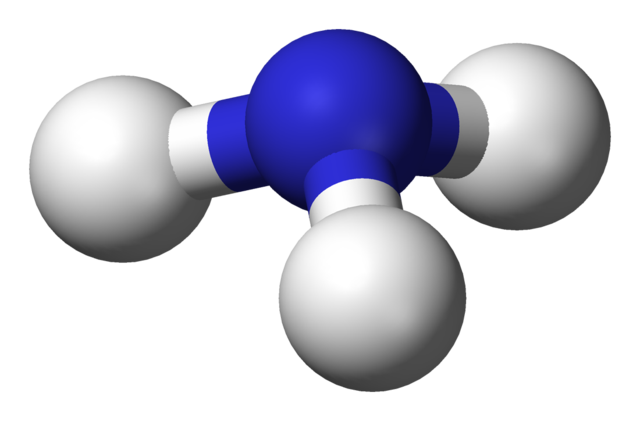1. When methane reacts with steam the temperature falls.

The equation for the reaction is:

CH4 + H2OCO + 3H2

What does the symbol represent, and is the reaction between methane and steam exothermic or endothermic?

 meaning of ⇌ Reaction type A equilibrium endothermic B equilibrium exothermic C Reversible reaction endothermic D Reversible reaction exothermic

Q2+3:
The following reversible reaction was investigated:

NH4Cl(s)NH3(g)  +  HCl(g)

2. What conditions are required to make this reaction go in the forward and the reverse directions?

 Forward Reverse A heat cool B heat heat C cool cool D cool heat
3. What type of reaction is the forward reaction?
• A.   neutralization
• B.   precipitation
• C.   sublimation
• D.   thermal decomposition
4. Which one of the following statements is true about reversible reactions?
• A. All reactions are reversible under lab conditions
• B. All reversible reactions are in dynamic equilibrium
• C. All reactions go to completion under lab conditions
• D. The direction of some reversible reactions can be altered by changing the reaction conditions

Q5+6:
Reversible reactions that are in dynamic equilibrium have two characteristics.

5. One of the characteristics is that the ..
• A.   forward reaction is faster than the reverse reaction
• B.   forward reaction is slower than the reverse reaction
• C.   forward and reverse reactions both stop
• D.   forward and reverse reactions occur at the same rate
6. The second characteristic is that the ..
• A.   concentrations of reactants and products are equal
• B.   concentrations of reactants and products are constant
• C.   concentration of reactants is less than concentration of products
• D.   concentration of reactants is more than concentration of products
7. Hydrated copper(II)sulfate can be converted to anhydrous copper(II)sulfate by heating strongly. The reaction is reversible:

CuSO4.5H2O(s)CuSO4(s)   +   5H2O(g)

The forward reaction is endothermic and absorbs 78.2 kJ/mol.

Which statement is true about the reverse reaction?

• A.   It is exothermic and releases less than 78.2kJ/mol
• B.   It is exothermic and releases 78.2kJ/mol
• C.   It is also endothermic and absorbs more than 78.2kJ/mol
• D.   It is also endothermic and absorbs 78.2kJ/mol

Q8-12:
Ammonia is produced industrially in the Haber Process.
The forward reaction is exothermic.

N2(g) + 3H2(g)2NH3(g)8. What gases will be present at equilibrium?
• A.   nitrogen and hydrogen only
• B.   ammonia only
• C.   nitrogen, hydrogen and ammonia
• D.   hydrogen only
9. What happens to the rate of reaction when the temperature is increased in the Haber Process?
10. What happens to the position of equilibrium when the temperature is increased in the Haber Process?
11. What happens to the rate of reaction when the pressure is decreased in the Haber Process?
12. What happens to the position of equilibrium when the pressure is decreased in the Haber Process?